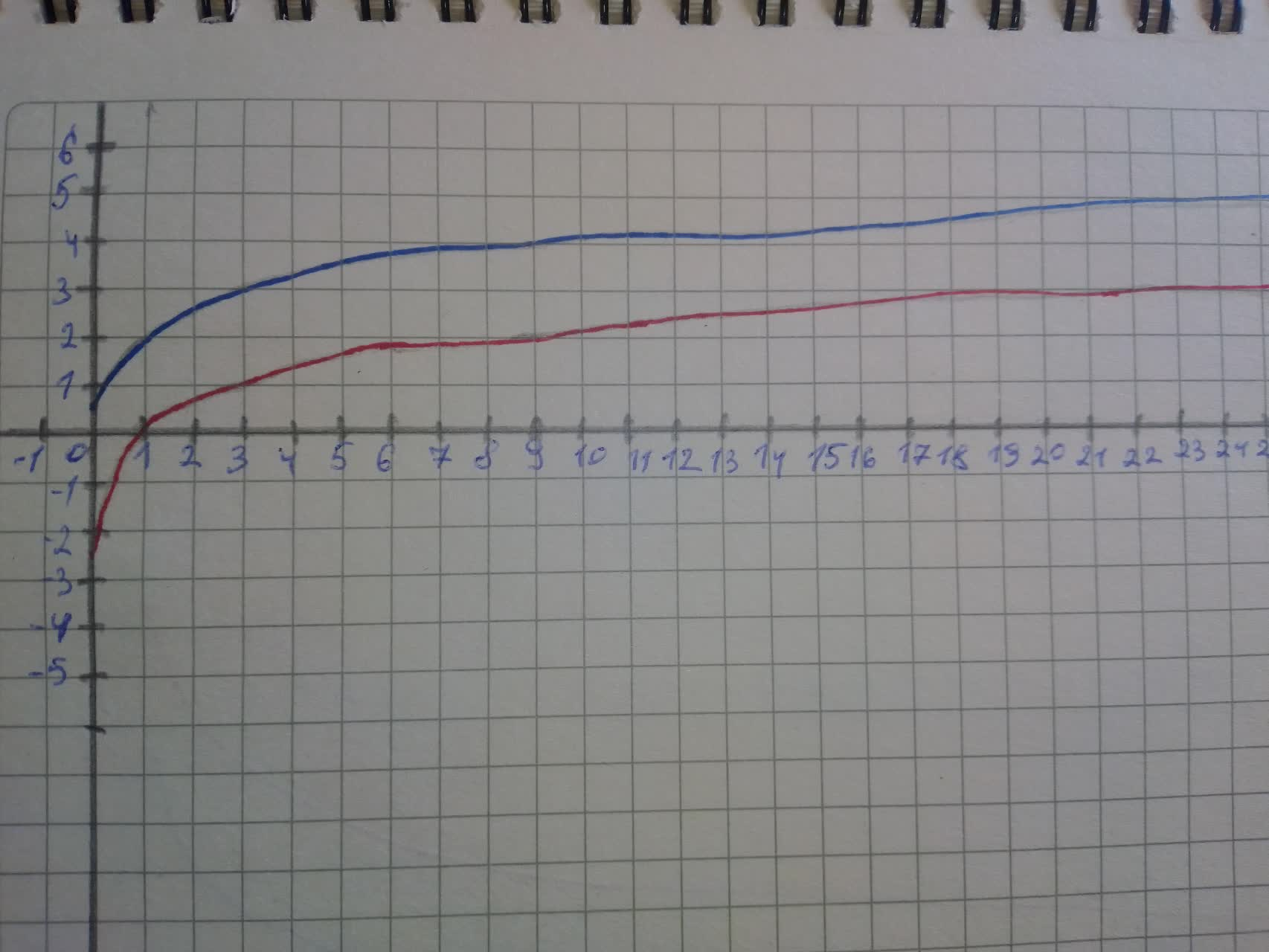Question# For y=2+ \log_{3}x. a) Use transformations of the graphs of y=\log_{2}x

Transformations of functions
ANSWEREDFor $$\displaystyle{y}={2}+{{\log}_{{{3}}}{x}}$$.
a) Use transformations of the graphs of $$\displaystyle{y}={{\log}_{{{2}}}{x}}\ {\quad\text{and}\quad}\ {y}={{\log}_{{{3}}}{x}}$$ o graph the given functions.
b) Write the domain and range in interval notation.
c) Write an equation of the asymptote.2021-08-15
Step 1
a)Start from the graph of the parent function $$\displaystyle{f{{\left({x}\right)}}}={{\log}_{{{3}}}{x}}$$
As we can see the given function $$\displaystyle{y}={{\log}_{{{3}}}{x}}+{2}$$ can be expressed in terms of the parent function f as $$\displaystyle{y}={f{{\left({x}\right)}}}+{2}$$
This indicates that the graph of the function $$\displaystyle{y}={{\log}_{{{3}}}{x}}+{2}$$ will be the same as the graph of the parent function $$\displaystyle{f{{\left({x}\right)}}}={{\log}_{{{3}}}{x}}$$ shifted 2 units upward.
See the graphs in the picture below:Step 2
b) The domain of the function $$\displaystyle{y}={{\log}_{{{3}}}{x}}+{2}$$ is the interval: $$\displaystyle{\left({0},+\infty\right)}$$
The range of the function $$\displaystyle{y}={{\log}_{{{3}}}{x}}+{2}$$ is the interval $$\displaystyle{\left(-\infty,+\infty\right)}$$
c) The vertical asymptote of the graph of this function is the line $$\displaystyle{x}={0}$$